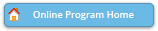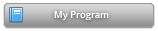#### Abstract Details

 Activity Number: 277 - Statistical Methods for Composite Time-To-Event Endpoints Type: Invited Date/Time: Tuesday, July 30, 2019 : 8:30 AM to 10:20 AM Sponsor: Lifetime Data Science Section Abstract #300567 Title: A Class of Proportional Win Fractions Regression Models for Composite Endpoints Author(s): Lu Mao* Companies: University of Wisconsin-Madison Keywords: cardiovascular trial; estimating equation; efficiency; semi-competing risks; survival analysis; win ratio Abstract: We propose a class of semiparametric regression models for composite endpoints based on a user-defined win function. The key assumption is that, given a pair of covariate-specified subgroups, the conditional probabilities of winning for each subgroup remain proportional over time. Under this assumption, the regression parameter can be interpreted as the log win ratio between two subgroups demarcated by one unit increase of the covariate. With suitable choices of the win function, the model yields a natural extension of the win-loss methods from two-sample testing to regression, or reduces to the familiar proportional hazards model for time to the first event. To make inference on the regression parameter, we construct a class of weighted estimating equations and investigate the efficiency of the resulting estimators with regard to the choice of the weight. We also develop a set of graphical and numerical tools to assess the plausibility of proportionality, among other model assumptions. Data from a major cardiovascular clinical trial are analyzed using the proposed methods as an illustration.

Authors who are presenting talks have a * after their name.